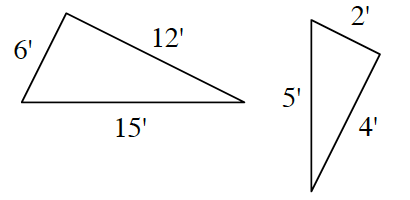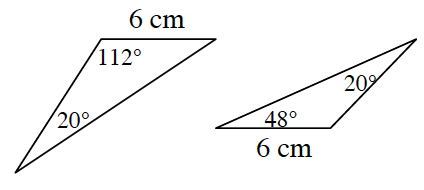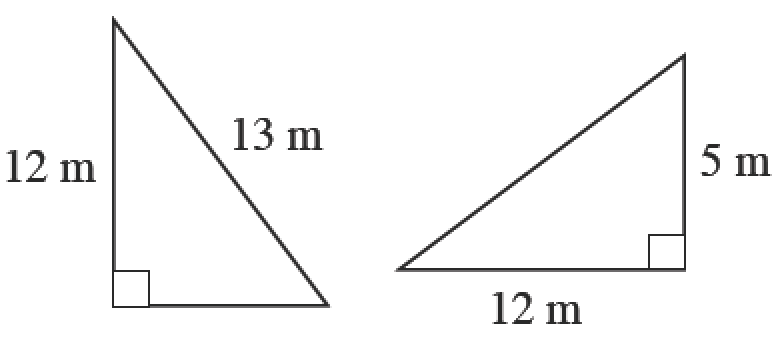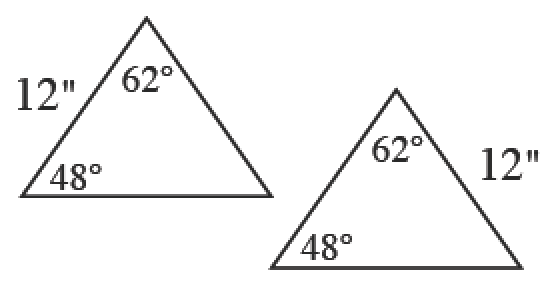### Home > CCG > Chapter 7 > Lesson 7.2.1 > Problem7-59

7-59.

Which pairs of triangles below are congruent and/or similar? For each part, explain how you know using an appropriate triangle congruence or similarity condition. Note: The diagrams are not necessarily drawn to scale.

What does it mean for two triangles to be congruent? What does it mean for two triangles to be similar?
How are these two definitions different?

1.Divide each side of the triangle on the left by $3$.
What do you notice?

1.congruent ($\text{ASA}≅$ or $\text{AAS}≅$)

1.Use the Pythagorean Theorem to solve for the missing side.

1.Where is the $12$ located on each triangle?

similar ($AA^{\sim}$) but not congruent since the two sides of length $12$ are not corresponding